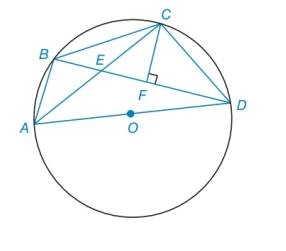Chapter 6.2, Problem 49E### Elementary Geometry for College St...

6th Edition
Daniel C. Alexander + 1 other
ISBN: 9781285195698

#### Solutions

Chapter
Section### Elementary Geometry for College St...

6th Edition
Daniel C. Alexander + 1 other
ISBN: 9781285195698
Textbook Problem
1 views

# In the figure, quadrilateral A B C D is inscribed in ⊙ O . Also, C F ¯ ⊥ B D ¯ .a) Explain why Δ A B E ∼ Δ D F C .b) Explain why ∠ D A B and ∠ B C D are supplementary.To determine

To explain:

The reason that ΔABEΔDFC.

Explanation

Calculation:

Given the figure,

From the figure, consider the below triangles ΔABE and ΔDFC.

To determine

To explain:

The reason that DAB and BCD are supplementary.

### Still sussing out bartleby?

Check out a sample textbook solution.

See a sample solution

#### The Solution to Your Study Problems

Bartleby provides explanations to thousands of textbook problems written by our experts, many with advanced degrees!

Get Started

#### Expand each expression in Exercises 122. (4y2)y

Finite Mathematics and Applied Calculus (MindTap Course List)

#### Sometimes, Always, or Never: If c is a critical number, then f′(c) = 0.

Study Guide for Stewart's Single Variable Calculus: Early Transcendentals, 8th

#### True or False: If and , then .

Study Guide for Stewart's Multivariable Calculus, 8th

#### Simplify each expression. 323/5

College Algebra (MindTap Course List)